# Deformation in Timber Under Load

This article is concerned with the type and magnitude of the deformation that results from the application of external load. As in the case of both concrete and polymers, the load–deformation relationship in timber is exceedingly complex, resulting from the facts that:

• timber does not behave in a truly elastic mode, rather its behaviour is time­dependent
• the magnitude of the strain is influenced by a wide range of factors; some of these are property dependent, such as density of the timber, angle of the grain relative to direction of load application, and angle of the microfibrils within the cell wall; others are environmentally dependent, such as temperature and relative humidity.

Under service conditions timber often has to withstand an imposed load for many years, perhaps even centuries; this is particularly relevant in construction applications. When loaded, timber will deform and a generalised interpretation of the variation in deformation with time together with the various components of this deformation is illustrated in Fig. 1.

The instantaneous (and reversible) deformation on load application at time t0 is true elastic behaviour. On maintaining the load to time tl the deformation increases by creep, although the rate of increase is continually decreasing. On load removal at time t1 an instantaneous recovery is approximately equal in magnitude to the initial elastic deformation. With time, the remaining deformation will decrease at an ever­decreasing rate until at time t2 no further reduction occurs.

The creep that has occurred during loading can be conveniently subdivided into a reversible component, which disappears with time and which can be regarded as delayed elastic behaviour, and an irreversible component, which results from plastic or viscous flow. Therefore, timber on loading possesses three forms of deformation behaviour – elastic, delayed elastic and viscous.

Like so many other materials, timber can be treated neither as a truly elastic material where, by Hooke’s law, stress is proportional to strain but independent of the rate of strain, nor as a truly viscous liquid where, according to Newton’s law, stress is proportional to rate of strain, but independent of strain itself. Therefore timber is, like many other materials, viscoelastic. Having defined timber as such (i.e. as a viscoelastic material), you will no doubt be surprised to find that half of what follows in this article is devoted to the elastic behaviour of timber.

The following section will indicate how, at low levels of stressing and short periods of time, there is considerable justification for treating the material as such. Perhaps the greatest incentive for this viewpoint is the fact that classical elasticity theory is well established and, when applied to timber, has been shown to work very well. The question of time in any stress analysis can be accommodated by the use of safety factors in design calculations.

Consequently, we will deal first with elastic deformation as representing a very good approximation of what happens in practice, and then follow this with viscoelastic deformation, which embraces both delayed elastic and irreversible deformation. Although technically more applicable to timber, viscoelasticity is certainly less well understood and developed in its application than is the case with elasticity theory.

## Elastic Deformation

When a sample of timber is loaded in tension, compression or bending, the instantaneous deformations are approximately proportional to the values of the applied load. Figure 2 illustrates that this approximation is certainly truer of the experimental evidence in longitudinal tensile loading than in the case of longitudinal compression.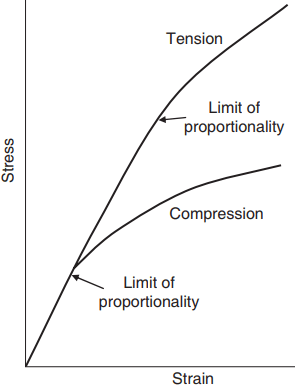Fig. 2 Stress–strain graphs for timber stressed in tension and compression parallel to the grain. The assumed limit of proportionality for each graph is indicated.

In both modes of loading, the approximation appears to become a reality at the lower levels of loading. The point of inflection of the stress–strain curve, i.e. the limit of propor tionality, is analogous to the yield or proof stress of metals.

Generally, the limit of proportionality in longitudinal tension is found to occur at about 60% of the ultimate load to failure, while in longitudinal compression the limit is considerably lower, varying from 30 to 50% of the failure value. As with other materials, at stresses below the limit of proportionality we can describe the behaviour with the modulus of elasticity (E), which is the slope of the straight line.

It is worth recalling that E is also referred to as the elastic modulus, Young’s modulus, or simply and frequently, though incorrectly, as stiffness. The apparent linearity at the lower levels of loading is really an artefact introduced by the rate of testing. At fast rates of loading, a very good approximation to a straight line occurs, but as the rate of loading decreases, the stress–strain line assumes a curvilinear shape (Fig. 3).

Such curves can be treated as linear by introducing a straight line approximation, which can take the form of either a tangent or a secant, giving the tangent or secant modulus.

This is common practice for timber and wood-fibre composites, with the tangent modulus determined at zero stress and the lower stress level for the secant modulus being zero (Fig. 3). Thus, while in theory it should be possible to obtain a true elastic response, in practice this is rarely the case, though the degree of divergence is frequently very low. It should be appreciated in passing that a curvilinear stress–strain curve must not be interpreted as an absence of true elastic behaviour.

The material may still behave elastically, though not linearly elastically; the prime criterion for elastic behaviour is that the stress–strain curve is truly reversible, i.e. no permanent deformation occurs on release of the load. The modulus of elasticity or elastic modulus in the longitudinal direction is one of the principal elastic constants of our material. You can find information on the different methods used to determine the value of the modulus under both static and dynamic loading in Dinwoodie (2000).

The elastic behaviour of a material is characterised by two further constants:

• the modulus of rigidity or the shear modulus, G – the slope of the shear stress vs. shear strain graph
• the Poisson’s ratio, ν – the ratio of the strain in the direction perpendicular to the applied stress to that in the direction of the stress.

Because of timber’s anisotropic behaviour and its treatment as a rhombic system, six Poisson’s ratios occur.

## Orthotropic Elasticity and Timber

In applying the elements of orthotropic elasticity to timber, the assumption is made that the three principal elasticity directions coincide with the longitudinal, radial and tangential directions in the tree. The assumption implies that the tangential faces are straight and not curved, and that the radial faces are parallel and not diverging.

However, by dealing with small pieces of timber removed at some distance from the centre of the tree, the approximation of rhombic symmetry for a system possessing circular symmetry becomes more and more acceptable. The nine independent constants required to specify the elastic behaviour of timber are the three moduli of elasticity, one in each of the longitudinal (L), radial (R) and tangential (T) directions; the three moduli of rigidity, one in each of the principal planes longitudinal–tangential (LT), longitudinal– radial (LR) and tangential–radial (TR); and three Poisson’s ratios, namely νRT, νLR and νTL.

Comparison of EL with either ER or ET, and GTR with GLT or GLR will indicate a degree of anisotropy that can be as high as 60:1; usually the ratio of EL to EHORIZ is of the order of 40:1. Note should be taken that the values of νTR are frequently greater than 0.5.

## Factors Influencing the Elastic Modulus

The elastic modulus of timber is influenced by many factors, some of which are properties of the material while others are components of the environment.

Grain angle: Figure 4, in addition to illustrating the marked influence of grain angle on elastic modulus, shows the degree of fit between experimentally derived values and the line obtained using transformation equations to calculate theoretical values.

Density: Elastic modulus is related to the density of timber, a relationship that is confirmed by the plot of over two hundred species of timber contained in Bulletin 50 of the former Forest Products Research Laboratory (Fig. 5); the correlation coefficient was 0.88 for timber at 12% moisture content and 0.81 for green timber, and the relation is curvilinear.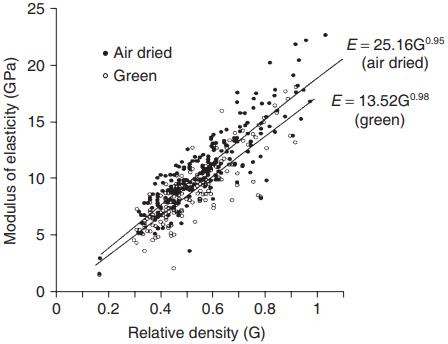Fig. 5 Effect of specific gravity on the longitudinalmodulus of elasticity for over 200 species of timbertested in the green and dry states.

A high correlation is to be expected, since density is a function of the ratio of cell wall thickness to cell diameter; consequently, increasing density will result in increasing elastic modulus of the cell. Owing to the variability in structure that exists between different timbers, the relationship between density and elastic modulus will be higher where only a single species is under investigation. Because of the reduced range in density, a linear regression is usually fitted.

Similar relationships with density have been recorded for the modulus of rigidity in certain species; in others, however, for example spruce, both the longitudinal–tangential and longitudinal–radial shear moduli have been found to be independent of density. Most investigators agree, however, that the Poisson’s ratios are independent of density.

Knots: Since timber is anisotropic in behaviour, and since knots are characterised by the occurrence of distorted grain, it is not surprising to find that the presence of knots in timber results in a reduction in the elastic modulus.

The relationship is difficult to quantify since the effect of the knots will depend not only on their number and size, but also on their distribution both along the length of the sample and across the faces. Dead knots, especially where the knot has fallen out, will result in larger reductions in elastic modulus than will green knots.

Ultrastructure: Two components of the fine or chemical structure have a profound influence on both the elastic and rigidity moduli. The first relates to the existence of a matrix material with particular emphasis on the presence of lignin. In those plants devoid of lignin, e.g. the grasses, or in wood fibres that have been delignified, the stiffness of the cells is low and it would appear that lignin, apart from its hydrophilic protective role for the cellulosic crystallites, is responsible to a considerable extent for the high elastic modulus found in timber.

The fact that lignin is significant determining the elastic modulus does not imply that the cellulose fraction plays no part; on the contrary, it has been shown that the angle at which the microfibrils are lying in the middle layer of the secondary cell wall, S2, also plays a significant role in controlling the elastic modulus (Fig. 6).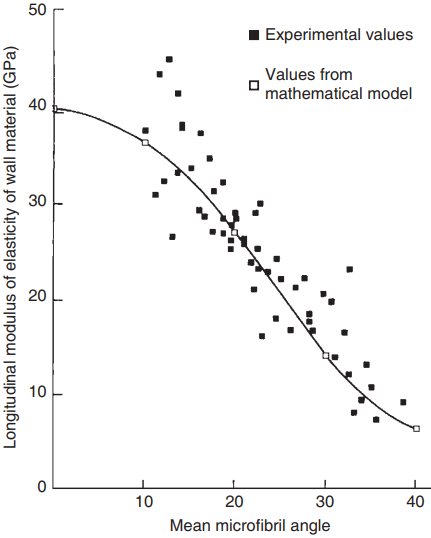Fig. 6 Effect of the mean microfibrillar angle of thecell wall on the longitudinal modulus of elasticity ofthe wall material in Pinus radiata. Calculated valuesfrom a mathematical model are also included.

A considerable number of mathematical models have been devised over the years to relate elastic modulus to microfibrillar angle. The early models were two­dimensional in approach, treating the cell wall as a planar slab of material, but later the models became much more sophisticated, taking into account the existence of cell-wall layers other than the S2, the variation in microfibrillar angle between the radial and tangential walls and consequently the probability that they undergo different strains, and, lastly, the possibility of complete shear restraint within the cell wall.

These three-­dimensional models are frequently analysed using finite element techniques. The early models were reviewed by Dinwoodie (1975). Later modelling of the behaviour of timber in terms of its structure has been reviewed by Astley et al. (1998); it makes reference to the work of Cave (1975), who used the concept of an elastic fibre composite consisting of an inert fibre phase embedded in a water­reactive matrix.

The constitutive equation is related to the overall elastic modulus of the composite, the volume fraction, and the elastic modulus and sorption characteristics of the matrix.

Unlike previous models, the equation can be applied not only to elasticity, but also to shrinkage and even moisture-induced creep. Recently, Harrington et al. (1998) have developed a model of the wood cell wall based on the homogenisation first, of an amorphous lignin–polyose matrix, and then of a representative volume element comprising a cellulose microfibril, its polyose–cellulose sheath and the surrounding matrix. The model predicts orthotropic elastic properties that are in good agreement with recorded values (Astley et al., 1998).

The predicted variation of axial elastic modulus with the S2 microfibrillar angle is consistent with observed behaviour and aligned with results from other cell wall models. The elastic modulus of a material is very dependent on the type and degree of chemical bonding within its structure; the abundance of covalent bonding in the longitudinal plane and hydrogen bonding in one of the transverse planes contributes considerably to the moderately high levels of elastic modulus characteristic of timber.

Moisture content: The influence of moisture content on elastic modulus is similar to, though not quite as sensitive as, that for strength. Early experiments in which elastic modulus was measured on a specimen of Sitka spruce as it took up moisture from the dry state clearly indicated a linear loss in modulus as the moisture content increased to about 27%, corresponding to the fibre saturation point; further increase in moisture content has no influence on the elastic modulus. These results for the variation in longitudinal moduli with moisture content under static loading have been confirmed using simple dynamic methods.

Measurement of the frequency of vibration – from which the moduli can be deduced – was carried out at regular intervals as samples of Sitka spruce were dried from 70% to zero moisture content (Fig. 7).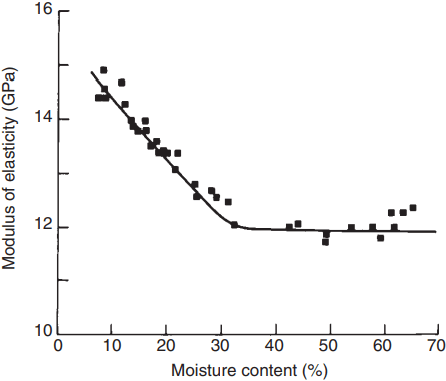Fig. 7 Effect of moisture content on thelongitudinal modulus of elasticity and the modulus ofrigidity in the L–R plane in Sitka spruce. Both moduliwere determined dynamically.

Confirmation of the reduction in modulus of elasticity with increasing moisture content is forthcoming first, from Fig. 5, in which the regression lines of elasticity against density for over 200 species of timber at 12% moisture content and in the green state are presented, and second, from the review of more recent results by Gerhards (1982).

Moisture increase has a far greater effect on the modulus perpendicular to the grain than on the modulus along the grain (Gerhards, 1982).

Temperature: In timber, like most other materials, increasing temperature results in greater oscillatory movement of the molecules and an enlargement of the crystal lattice. These in turn affect the mechanical properties, and the elastic modulus and strength of the material decrease.

Although the relationship between modulus and temperature has been shown experimentally to be curvilinear, the degree of curvature is usually slight at low moisture contents and the relation is frequently treated as linear thus:

ET = Et[1 – a(T – t)]

where E is the elastic modulus, T is a higher temperature, t is a lower temperature, and a is the temperature coefficient. The value of a for the longitudinal modulus has been shown to lie between 0.001 and 0.007 for low moisture contents.

The effect of a temperature increase is greater on the perpendicular modulus than on the longitudinal modulus (Gerhards, 1982). At higher moisture contents the relationship between elastic modulus and temperature is markedly curvilinear and the interaction of moisture content and temperature in influencing modulus is clearly shown in Fig. 8.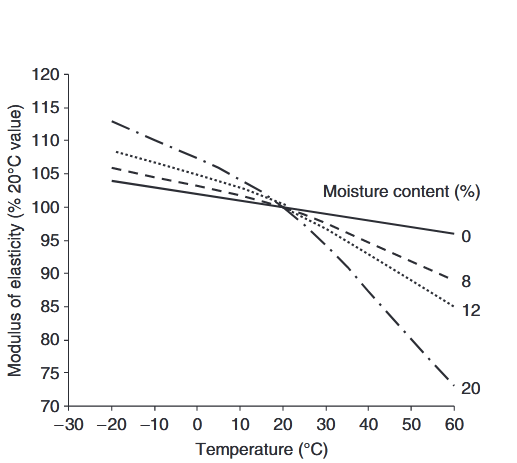Fig. 8 The interaction of temperature andmoisture content on the modulus of elasticity. Resultsare averaged for six species of timber and the modulusat 20ºC and 0% moisture content is taken as unity.

At zero moisture content the reduction in modulus between -20 and +60oC is only 6%; at 20% moisture content the corresponding reduction is 40%. This increase in the effect of temperature with increasing moisture content has been confirmed by Gerhards (1982). Long-term exposure to elevated temperature results in a marked reduction in elastic modulus as well as strength and toughness, the effect usually being greater in hardwoods than in softwoods.

Even exposure to cyclic changes in temperature over long periods will result in a loss of elastic modulus (Moore, 1984).